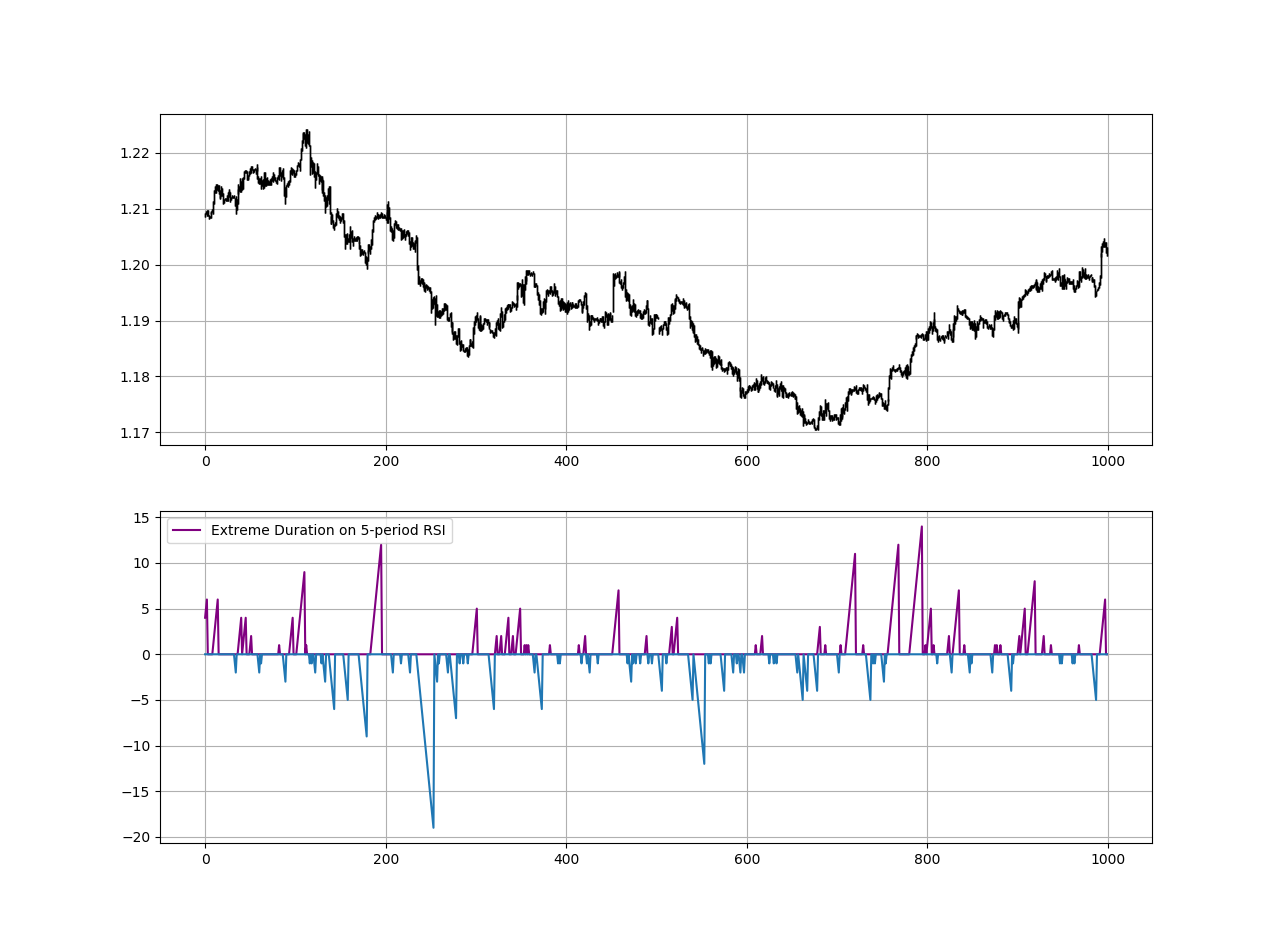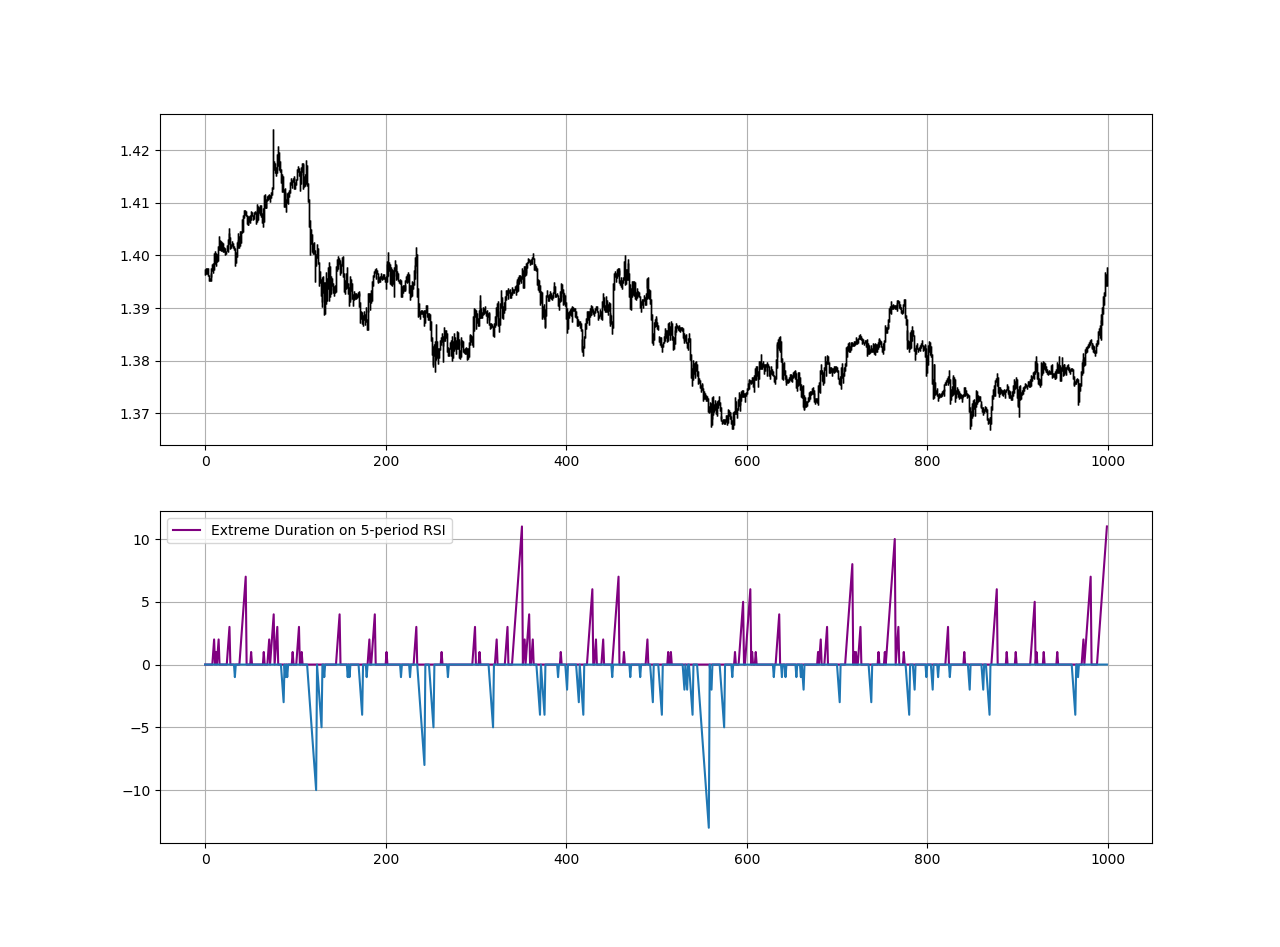# The Relative Strength Index

First introduced by J. Welles Wilder Jr., the RSI is one of the most popular and versatile technical indicators. Mainly used as a contrarian indicator where extreme values signal a reaction that can be exploited. Typically, we use the following steps to calculate the default RSI:

• Calculate the change in the closing prices from the previous ones.
• Separate the positive net changes from the negative net changes.
• Calculate a smoothed moving average on the positive net changes and on the absolute values of the negative net changes.
• Divide the smoothed positive changes by the smoothed negative changes. We will refer to this calculation as the Relative Strength — RS.
• Apply the normalization formula shown below for every time step to get the RSI.

The above chart shows the hourly values of the GBPUSD in black with the 13-period RSI. We can generally note that the RSI tends to bounce close to 25 while it tends to pause around 75. To code the RSI in Python, we need an OHLC array composed of four columns that cover open, high, low, and close prices.

`def adder(data, times):  for i in range(1, times + 1):  new = np.zeros((len(data), 1), dtype = float)  data = np.append(data, new, axis = 1) return datadef deleter(data, index, times):  for i in range(1, times + 1):  data = np.delete(data, index, axis = 1) return data def jump(data, jump):  data = data[jump:, ]  return datadef ma(data, lookback, close, where):   data = adder(data, 1)  for i in range(len(data)):  try:  data[i, where] = (data[i - lookback + 1:i + 1, close].mean())  except IndexError:  pass  data = jump(data, lookback)  return datadef ema(data, alpha, lookback, what, where):  alpha = alpha / (lookback + 1.0)  beta = 1 - alpha  data = ma(data, lookback, what, where)data[lookback + 1, where] = (data[lookback + 1, what] * alpha) + (data[lookback, where] * beta)for i in range(lookback + 2, len(data)):  try:  data[i, where] = (data[i, what] * alpha) + (data[i - 1, where] * beta)  except IndexError:  pass  return datadef rsi(data, lookback, close, where):  data = adder(data, 5)  for i in range(len(data)):  data[i, where] = data[i, close] - data[i - 1, close]  for i in range(len(data)):  if data[i, where] > 0:  data[i, where + 1] = data[i, where]  elif data[i, where] < 0:  data[i, where + 2] = abs(data[i, where])  lookback = (lookback * 2) - 1 # From exponential to smoothed data = ema(data, 2, lookback, where + 1, where + 3) data = ema(data, 2, lookback, where + 2, where + 4) data[:, where + 5] = data[:, where + 3] / data[:, where + 4]  data[:, where + 6] = (100 - (100 / (1 + data[:, where + 5]))) data = deleter(data, where, 6) data = jump(data, lookback) return data`

Medium is a hub to many interesting reads. I read a lot of articles before I decided to start writing. Consider joining Medium using my referral link!

# The Extreme Duration

I have presented the RSI first because the extreme duration is simply an add-on to any bounded indicator. It is not an indicator on its own per se, but simply a way to see how long the indicator spends being overbought or oversold. We understand that:

• An overbought indicator is saying that the bullish momentum should fade soon and we might see a correction or even a reversal on the downside.
• An oversold indicator is saying that the bearish momentum should fade soon and we might see a correction or even a reversal on the upside.

However, if things were so simple, we would all be millionaires. With this being said, let us take a look at the extreme duration below (calculated on the values of the RSI) before seeing how to code and analyze it.EURUSD in the first panel with the Extreme Duration on the second panel.

The above plot shows the extreme duration calculation based on a 5-period RSI with barriers at 70 and 30. When the Extreme Duration is showing a reading of 5, it means that the 5-period RSI is above 70 for the fifth consecutive time (i.e. surpassed 70 and stayed above it for 5 units of time). We are used to analyze these indicators value-wise, we want to analyze them this time time-wise. To calculate the extreme duration, we can follow the below steps:

`# Calculate the RSI on OHLC array (composed of 4 columns)my_data = rsi(my_data, 5, 3, 4)# Define the Barriers (Oversold and Overbought zones)upper_barrier = 70lower_barrier = 30# Define the Extreme Durationdef extreme_duration(data, indicator, upper_barrier, lower_barrier, where_upward_extreme, where_downward_extreme):  data = adder(data, 2) # Time Spent Overbought  for i in range(len(data)):   if data[i, indicator] > upper_barrier: data[i, where_upward_extreme] = data[i - 1, where_upward_extreme] + 1  else: a = 0 data[i, where_upward_extreme] = a   # Time Spent Oversold   for i in range(len(data)):  if data[i, indicator] < lower_barrier: data[i, where_downward_extreme] = data[i - 1, where_downward_extreme] + 1  else: a = 0 data[i, where_downward_extreme] = a  data[:, where_downward_extreme] = -1 * data[:, where_downward_extreme]  return data# Calculating the Extreme Durationmy_data= extreme_duration(my_data, 4, upper_barrier, lower_barrier, 5, 6)`GBPUSD in the first panel with the Extreme Duration on the second panel.

Let us now try to use the extreme duration to generate signals based on this hypothesis:

“What if the average time spent on the extremes can help us understand when the indicator will exist these mentioned extremes?”

Based on a historical analysis, the average duration of the 5-period RSI is between 3 and 4. However, based on visual historical representation, it is closer to 5. Let us set the time barriers to 5 and -5.

`def signal(data, upward_timing, downward_timing, buy, sell): data = adder(data, 2) for i in range(len(data)):  if data[i, downward_timing] <= -5 and data[i - 1, downward_timing] >= -5 and data[i - 2, downward_timing] >= -5: data[i, buy] = 1  if data[i, upward_timing] >= 5 and data[i - 1, upward_timing] <= 5 and data[i - 2, upward_timing] <= 5 : data[i, sell] = -1  return data`